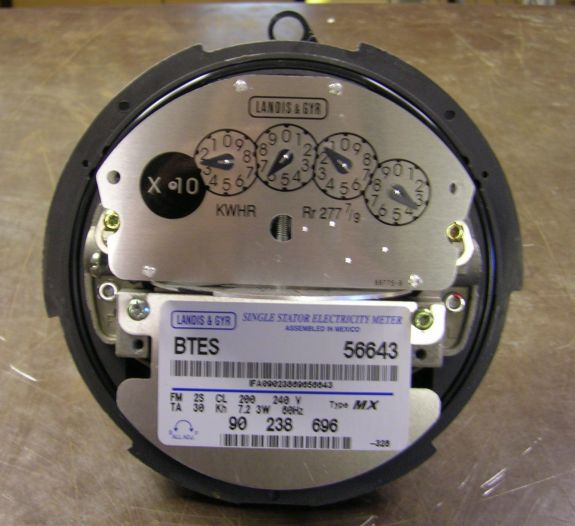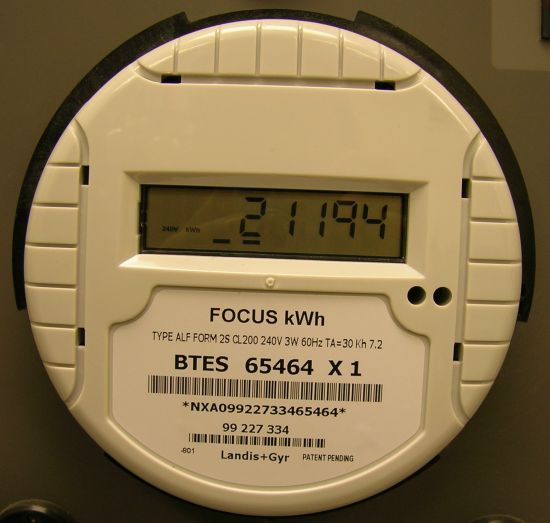BTES customers receive a bill every month based on the amount of electric usage that is used at their home or business. We obtain the amount of usage when we read your meter each month. This data is collected by a person or by a computer, depending on the type of meter that is on your house.If your meter is similar to the one shown on the right (click to view larger image), it is a “dial” meter. The faces of these meters have numbers 0 through 9 with 0 at the top. As you can see, some of the numbers run clockwise and others run counterclockwise. The hands of each dial move in the same direction as the numbers.

To read the meter, start with the left-hand dial and proceed right. If the hand is between two numbers, read the smaller number. As you can see, the meter shown reads 2613.

On the left side of this meter, you will notice a black circle that reads “X10.” If you subtract your last meter reading from your current meter reading and multiply that number by 10, you will get the number of kilowatt hours used since the last reading. A kilowatt hour is the amount of power it takes to burn ten 100-watt bulbs for one hour.

For example, the last reading on the above meter was 2449. Therefore we would subtract 2449 from 2613 and multiply the answer by 10 which will equal the number of kilowatt hours used:2613 – 2449 = 164
164 x 10 = 1,640 kWh

BTES also uses digital meters like the one shown on the right (click to view larger image). This meter reads 21194 and shows “X1.” Readings from this type of meter do not have a multiplier of 10. Therefore, to obtain the number of kilowatt hours used since the last reading, you simply subtract your last meter reading from your current meter reading.

BTES reads every customer meter every month. Our meter readers work hard to ensure that every meter is read correctly. They also check to make sure that the meters are working properly and look for damages and tampering. Tampering with your meter has serious consequences including criminal prosecution.

Not a BTES customer yet?